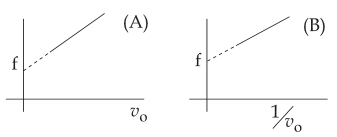#### A source of sound emits sound waves at frequency. It is moving towards an observer with fixed speedIf the observer were to move towards the source with speed, one of the following two graphs (A and B) will give the correct variation of the frequency f heard by the observer asis changed.The variation of f withis given correctly by : Option 1) graph A with slope  =Option 2) graph A with slope  =Option 3) graph B with slope  =Option 4)   graph B with slope  =As we learnt in

Frequency of sound when observer is stationary and source is moving towards observer -- whereinspeed of soundspeed of sourceoriginal frequencyapparent frequency

Graph A with slopeCorrect option is 1.

Option 1)

graph A with slope  =This is the correct option.

Option 2)

graph A with slope  =This is an incorrect option.

Option 3)

graph B with slope  =This is an incorrect option.

Option 4)

graph B with slope  =This is an incorrect option.

#### Plabita